## Single Machine Infinite Bus in Power System:

Figure 12.14 is the circuit model of a Single Machine Infinite Bus in Power System through a line of reactance Xe. In this simple case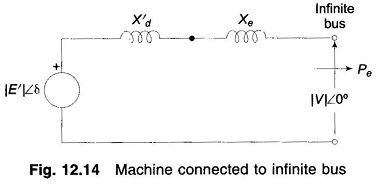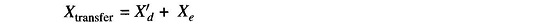From Eq. (12.29b)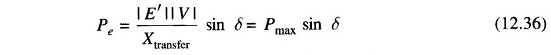The dynamics of this system are described in Eq. (12.11) as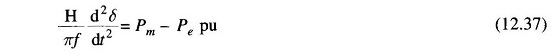### Two Machine System:

The case of two finite machines connected through a line (Xe) is illustrated in Fig. 12.15 where one of the machines must be generating and the other must be motoring.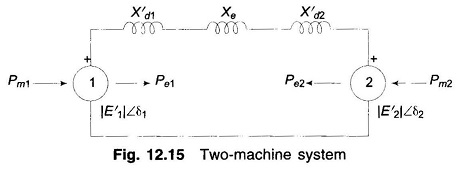Under steady condition, before the system goes into dynamics and the mechanical input/output of the two machines is assumed to remain constant at these values throughout the dynamics (governor action assumed slow). During steady state or in dynamic condition, the electrical power output of the generator must be absorbed by the motor (network being lossless). Thus at all time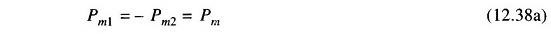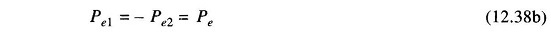The swing equations for the two machines can now be written as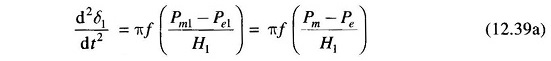and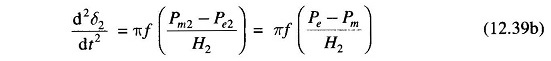Subtracting  Eq. (12.39b) from Eq. (12.39a)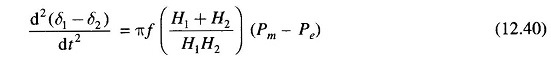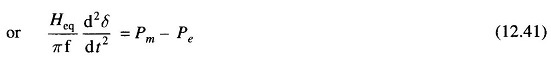where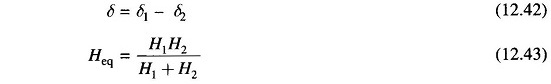The electrical power interchange is given by expression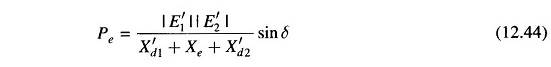The swing equation Eq. (12.41) and the power angle equation Eq. (12.44) have the same form as for a single machine connected to infinite bus. Thus a two-machine system is equivalent to a single machine connected to infinite bus.

Scroll to Top